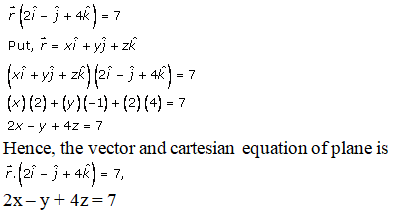# RD Sharma Solutions Class 12 The Plane Exercise 29.3

RD Sharma Solutions for Class 12 Maths Chapter 29 The Plane Exercise 29.3, is provided here. Our solution module utilizes various shortcut tips and practical examples to explain all the exercise questions in a simple and easily understandable language and to help students grasp the concepts faster and to make learning fun. The RD Sharma Solutions to this exercise are provided in pdf format, which can be downloaded easily from the links provided below.

## Download PDF of RD Sharma Solutions For Class 12 Maths Chapter 29 Exercise 3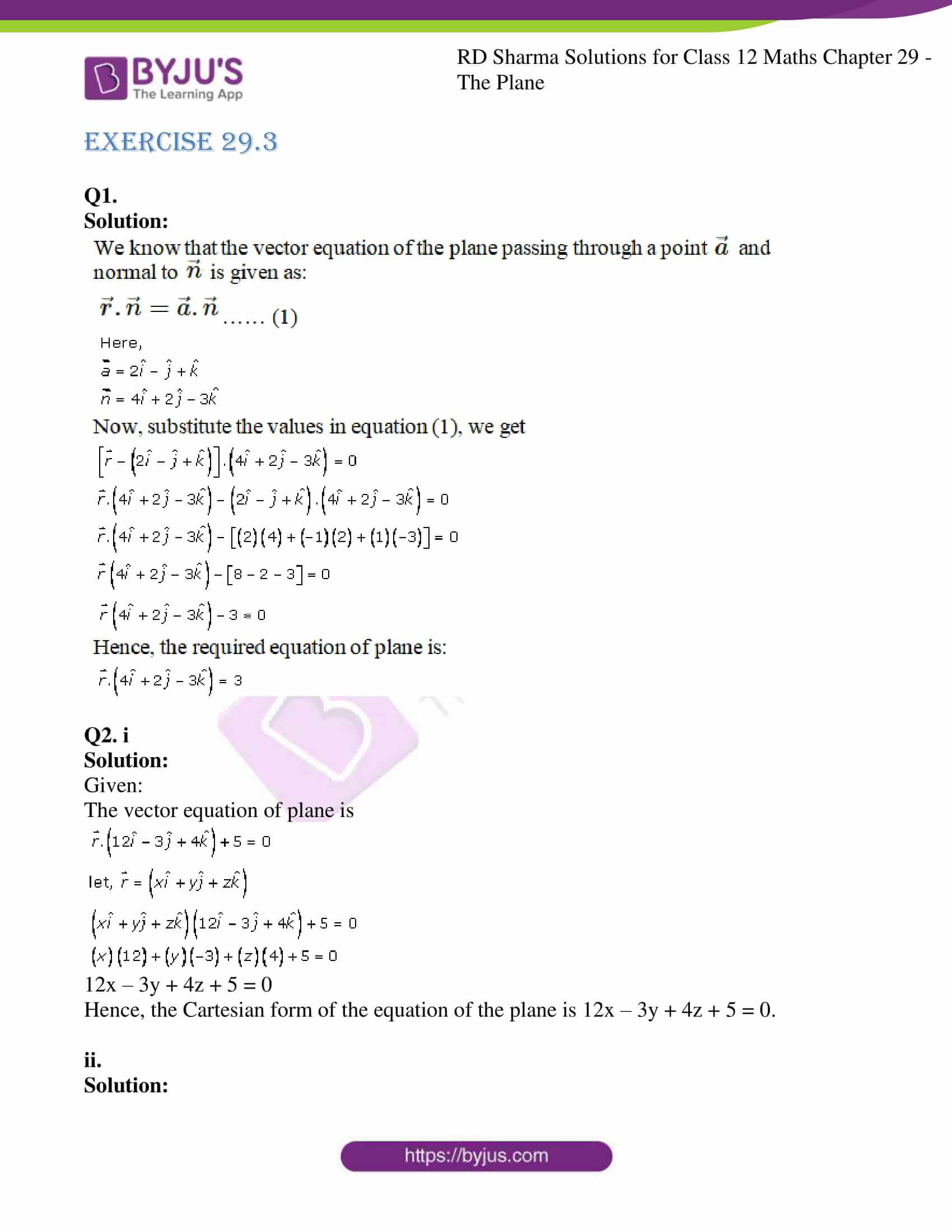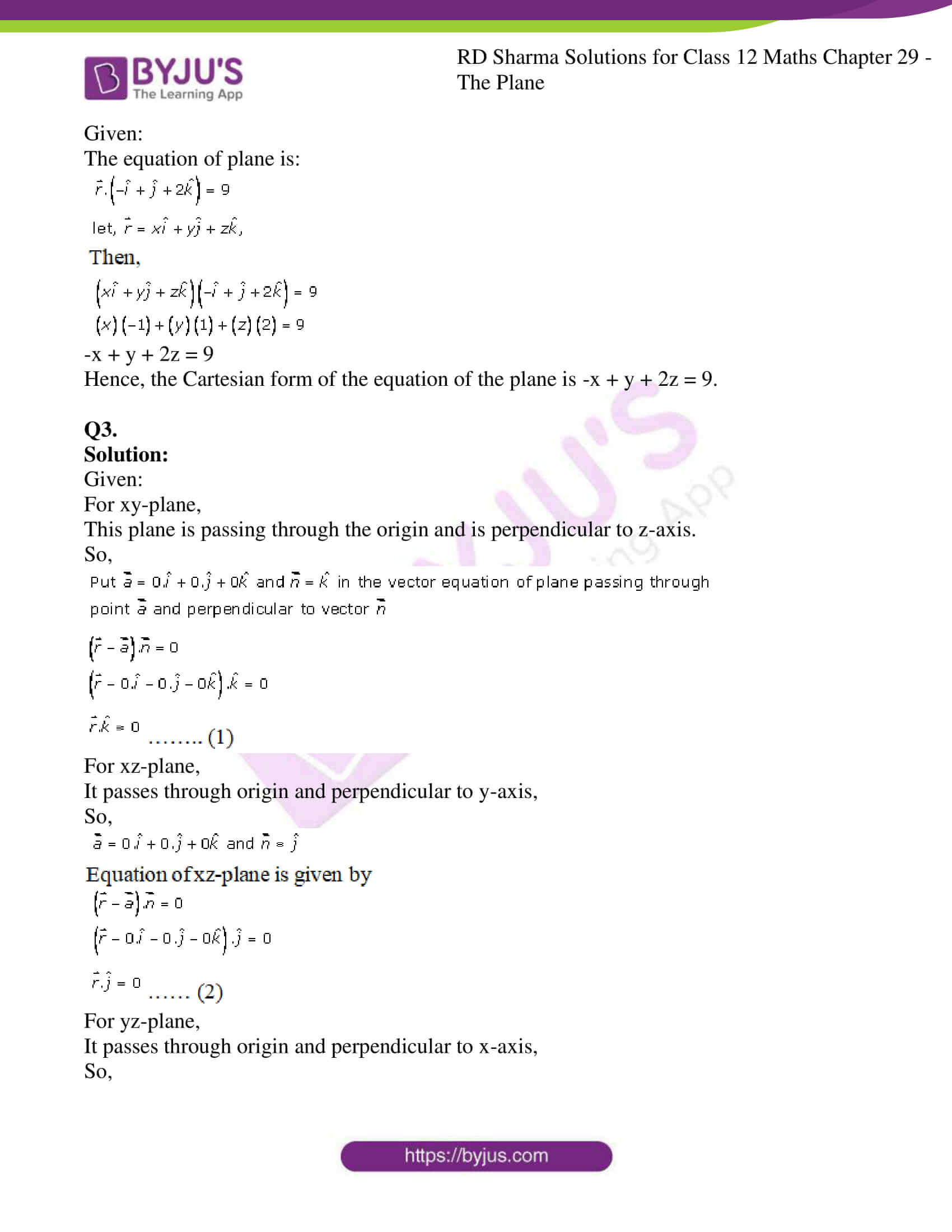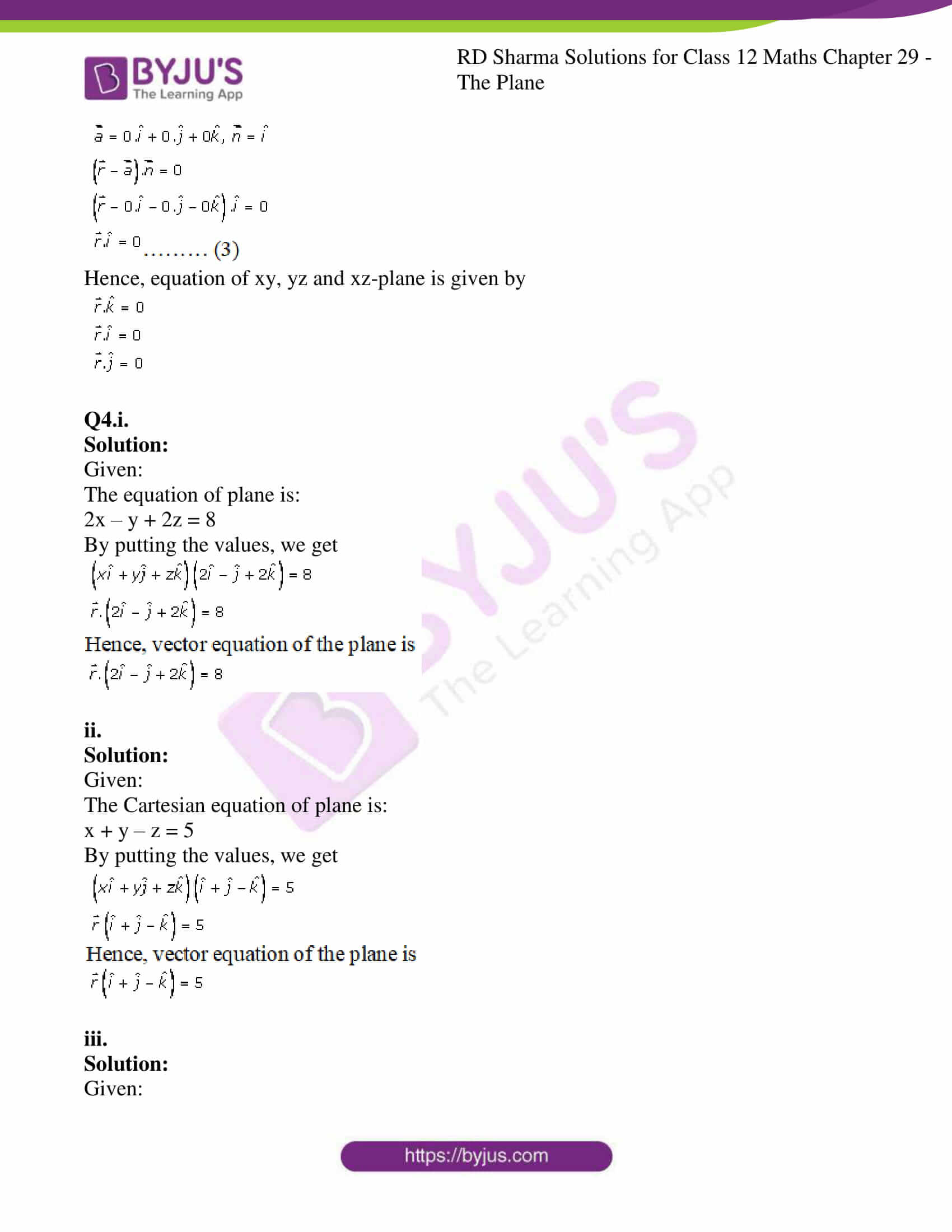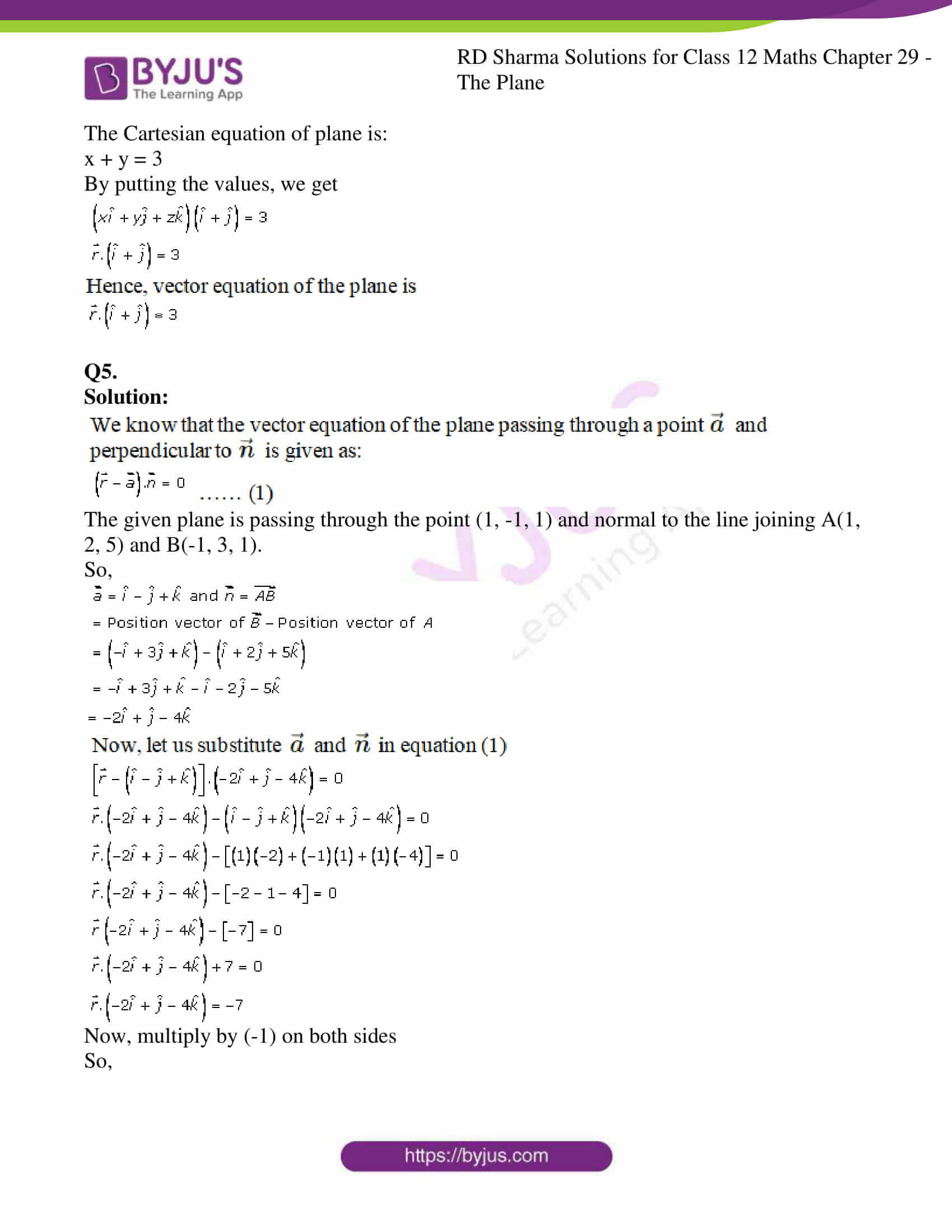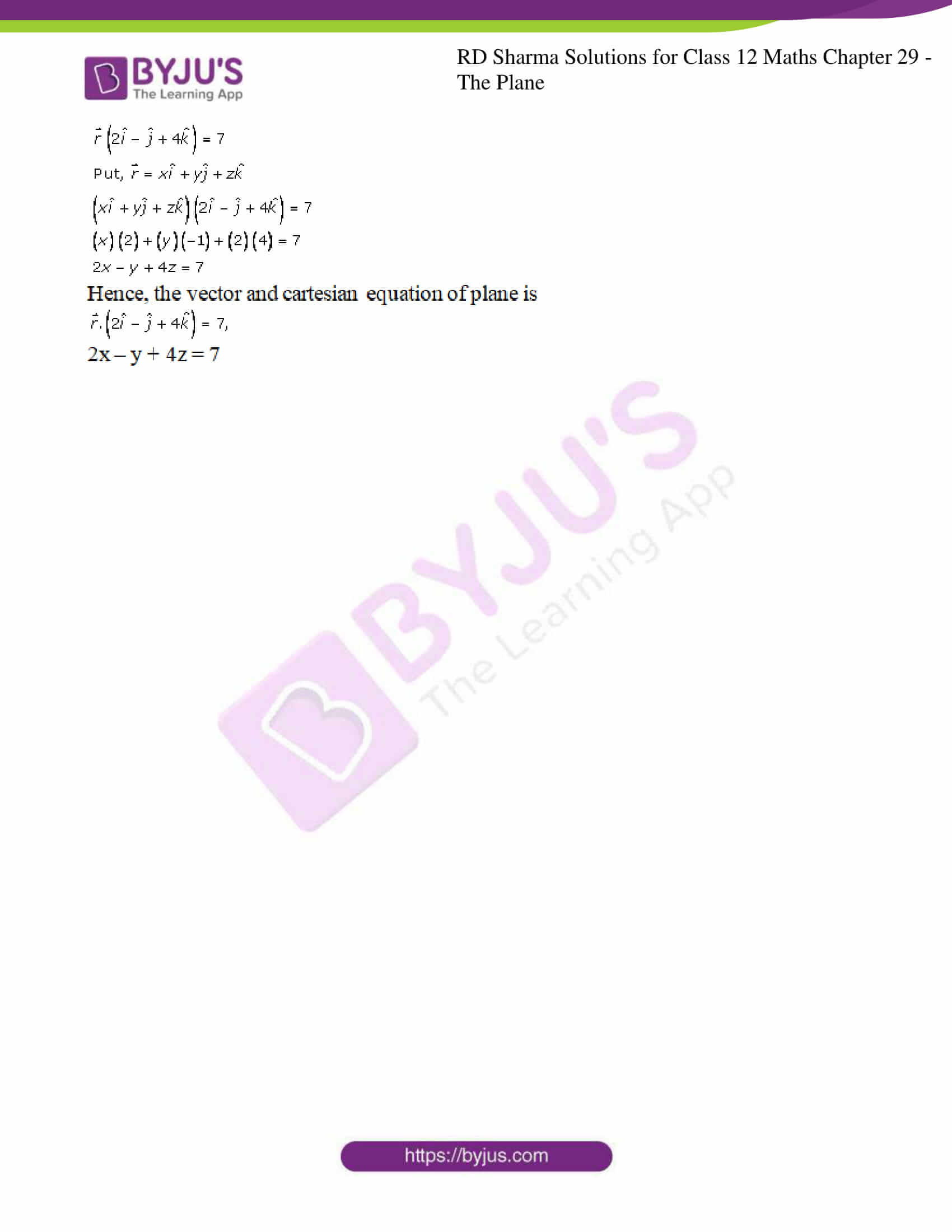### Access RD Sharma Solutions For Class 12 Maths Chapter 29 Exercise 3

EXERCISE 29.3

Q1.

Solution: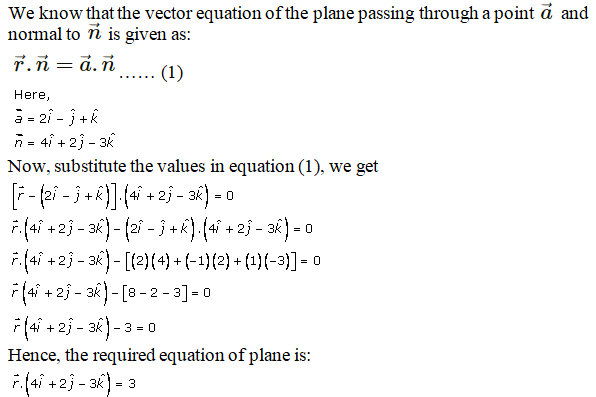Q2. i

Solution:

Given:

The vector equation of plane is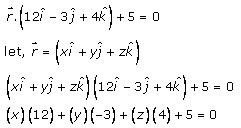12x – 3y + 4z + 5 = 0

Hence, the Cartesian form of the equation of the plane is 12x – 3y + 4z + 5 = 0.

ii.

Solution:

Given:

The equation of plane is: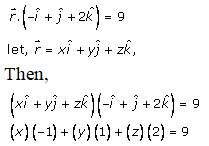-x + y + 2z = 9

Hence, the Cartesian form of the equation of the plane is -x + y + 2z = 9.

Q3.

Solution:

Given:

For xy-plane,

This plane is passing through the origin and is perpendicular to z-axis.

So,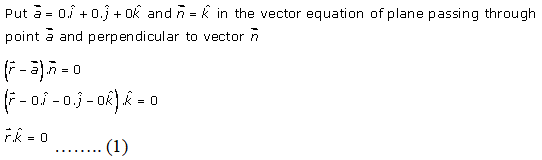For xz-plane,

It passes through origin and perpendicular to y-axis,

So,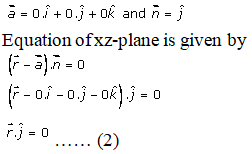For yz-plane,

It passes through origin and perpendicular to x-axis,

So,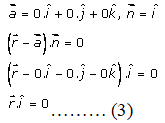Hence, equation of xy, yz and xz-plane is given by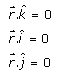Q4.i.

Solution:

Given:

The equation of plane is:

2x – y + 2z = 8

By putting the values, we get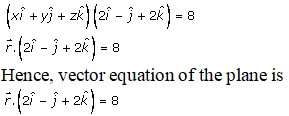ii.

Solution:

Given:

The Cartesian equation of plane is:

x + y – z = 5

By putting the values, we get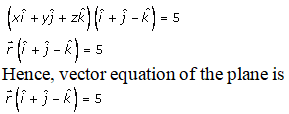iii.

Solution:

Given:

The Cartesian equation of plane is:

x + y = 3

By putting the values, we get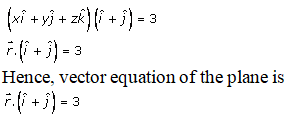Q5.

Solution: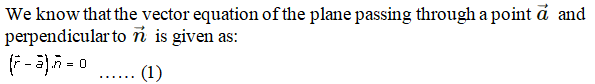The given plane is passing through the point (1, -1, 1) and normal to the line joining A(1, 2, 5) and B(-1, 3, 1).

So,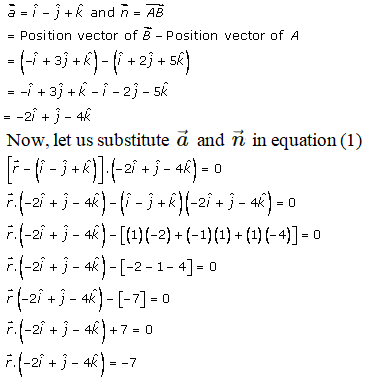Now, multiply by (-1) on both sides

So,